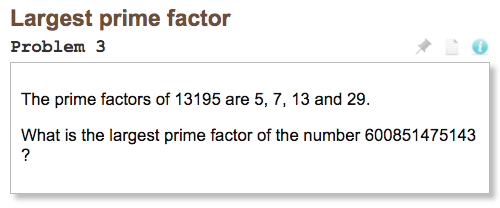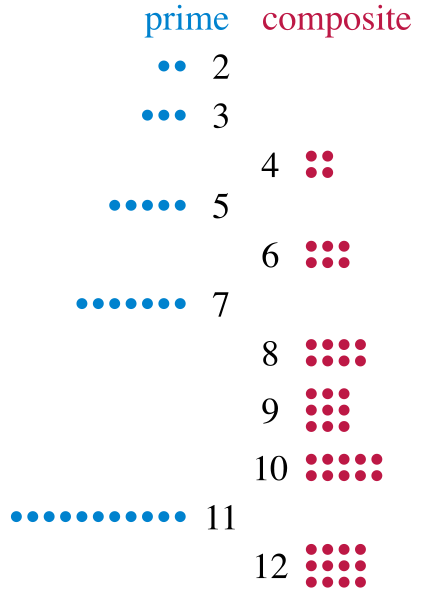# Project Euler Problem 3: Largest Prime FactorProblem 3 is where Euler starts forcing us to consider resource limitations. Before, the most straightforward solution worked just fine, even if it used more resources than a less complex algorithm would. As we’ll see here, that kind of solution, while correct doesn’t work for us because the program never finishes in a reasonable amount of time. If we’re going to solve this one we need to start thinking about algorithmic complexity.

As usual, this is a good chance to take some time with the problem yourself before continuing on.Project Euler really likes to play with prime numbers. Image: David Eppstein

## The Straightforward (Naive) Approach

So far I’ve been super intentional about saying “straightforward” when talking about the first solution that comes to mind. A lot of people call this the “naive” solution. I understand why people say “naive” but I usually avoid that word because I don’t like the negative connotations it carries. As we’ve seen, sometimes the “naive” or “straightforward” solution really is the best solution: it really depends on your context and the tradeoffs you’re making. I’ve seen people internalize the principle that the straightforward solution must be a “bad” solution and refuse to consider the tradeoffs involved. In the real world that mindset will just kill your productivity and lead you to produce work that isn’t appropriate for the context you’re working in.

That said, there are situations where it’s easier to call a solution “naive” and this problem gives us a good example. My first attempt at solving this problem went like this:

Ok, let’s just iterate over every number between 1 and 600,851,475,143, test whether it’s prime, then if so test whether it’s a factor of 600,851,475,143. Finally take the biggest one and there’s your answer.

In code that would look something like this. Ignore the magic is_prime() function for now, we’ll get to that further down:

factors = []
for x in range(2, 600851475143):
if is_prime(x):
if 600851475143 % x == 0:
factors.append(x)

print(factors[-1])


Now, it’s important to note that this solution is correct. We can test it on the example the problem gives us (13195) and we get the right answer (29). And we get it fast: running it on my machine there’s no noticeable delay. But when I plug in 600851475143 the program just seems to freeze. What gives? Even after waiting five minutes the program is just sitting there. What’s happening?

### Tinkering and timing

Let’s keep the logic of the program the same and play around with inputs to see if we get any hints about what the problem is. Remember I’m still using a magic is_prime() function that we’ll get to below.

Here we refactor our program into a function to make it easier to call repeatedly on different inputs:

def largest_prime_factor(n):
factors = []
for x in range(2, n):
if is_prime(x):
if n % x == 0:
factors.append(x)
return factors[-1]


Now we can easily run that code with multiple inputs:

>>> largest_prime_factor(13195)
29
>>> largest_prime_factor(21952)
7
>>> largest_prime_factor(98989)
8999


On my laptop, those first two examples seemed to run instantaneously, but the third one had a noticeable delay. Let’s measure how long the program takes to run every time we run it. We’ll use a simple way to time our code. There are of course, much more accurate ways to measure performance that are well suited for real-world profiling, but this is good enough for us right now.

import time
def timed (n):
start = time.clock()
result = largest_prime_factor(n)
end = time.clock()
duration = end - start
print('Largest prime factor of {} is {}. Execution: {} seconds'.format(
n, result, duration
))


Now we can see how fast the code is running:

>>> timed(13195)
"Largest prime factor of 13195 is 29. Execution: 0.018383000000000038 seconds"
>>> timed(21952)
"Largest prime factor of 21952 is 7. Execution: 0.026741000000015447 seconds"
>>> timed(98989)
"Largest prime factor of 98989 is 8999. Execution: 0.13390699999999356 seconds"


Of course, all these numbers are specific to my computer and setup. They’ll fluctuate a little each time I run the code, and they’ll also change depending on what else my computer is doing and several other factors. So if you run this you’ll get different numbers. That’s ok. The point is that I can easily see how execution time increases as I increase the size of the input.

That last part is important and bears repeating. Execution time increases as I increase the size of the input. Bigger inputs take more time to process. Thinking about the for loop in our largest_prime_factor function it makes a lot of sense: the more we loop the longer our program takes to run.

Let’s start adding zeros to our input and see what happens:

>>> timed(10000)
"Largest prime factor of 10000 is 5. Execution: 0.01425699999998642 seconds"
>>> timed(100000)
"Largest prime factor of 100000 is 5. Execution: 0.13481099999998492 seconds"
>>> timed(1000000)
"Largest prime factor of 1000000 is 5. Execution: 2.938510000000008 seconds"
>>> timed(10000000)
"Largest prime factor of 10000000 is 5. Execution: 74.51557199999999 seconds"


Uuuugh, that last one was a pain to wait for. And the rules of Project Euler say that solutions should run in a minute or less so that’s no good. Every time we add a zero to our input the code takes at least 10 times longer to run. The input we need to solve for, 600851475143, has 4 more digits than the number that took 74 seconds to solve. Back of the napkin, running our program on it will take something like $74 * 10^4 = 740{,}000$ seconds to run. That’s 8 or 9 days. No good.

## Correct isn’t Good Enough

If we’re actually going to solve this we need a better “solution”. It isn’t good enough that our solution is correct. The solution above is correct. But we don’t have the resources to actually run it on the input we need to so it’s as good as useless. In our context, the naive solution really is naive: it doesn’t take into account critical resource limitations.

Computer cycles are cheap. But they aren’t free. Give or take, my 2017 Macbook Air can run a simple python loop about $10{,}000{,}000$ times in a second. That’s:

• $\approx 1 * 10^7$ loops in a second
• $\approx 1 * 10^{14}$ loops in a year
• $\approx 1 * 10^{16}$ loops in a lifetime (~80 years)

Viewed through the lens of a human life, burning a million “Macbook loops” of time here or there doesn’t matter. But my computing resources are limited. As the exponent on a program’s computational needs increases that starts to matter more and more and more.

Let’s see if we can refactor our correct code into an actual, pragmatic solution.

## Correct and Efficient

Ok, so life is to short for programs that loop 600851475143 times. How can we find the factors of a number without needing so many loops?

The key insight for this problem is to see that factors come in pairs. Take an easy number like $2{,}500$. We can see right away that $2$ is a factor:

$$2{,}500 / 2 = 1{,}250$$

Hey look at that, we got another factor for free! If we see that $2$ is a factor we can deduce that $1,250$ must also be a factor. Let’s do this a few more times and see if any patterns stand out:

$$2{,}500 / 5 = 500$$ $$2{,}500 / 10 = 250$$ $$2{,}500 / 20 = 125$$ $$2{,}500 / 25 = 100$$ $$2{,}500 / 50 = 50$$

As one factor gets larger it’s “pair” factor gets smaller and smaller. Until they meet. Where do they meet? At the square root: $\sqrt(2{,}500)$ is $50$. That means every factor of $n$ larger than $\sqrt(n)$ is paired with a factor smaller than $\sqrt(n)$. And so: we can find every factor of $n$ just by looking at the integers up to $\sqrt(n)$!

We can use this to improve our solution. Instead of looping every number below 600851475143 to find factors we can just loop over every integer below its square root: about 775146. That’s… a lot fewer loops. In code:

import math
def largest_prime_factor(n):
factors = []
for x in range(2, math.floor(math.sqrt(n)) + 1):
if is_prime(x):
if n % x == 0:
pair = n / x
factors.append(x)
if is_prime(pair):
factors.append(pair)
return sorted(factors)[-1]


Ok, let’s check against our test input to see if it works:

>>> largest_prime_factor(13195)
29


Yep, still works. How fast?

>>> timed(13195)
"Largest prime factor of 13195 is 29. Execution: 0.0001859999999851425 seconds"


Hey, that’s a lot faster than our naive solution. Is this good enough?

>>> timed(600851475143)
"Largest prime factor of 600851475143 is [censored]. Execution: 2.0993389999999863 seconds"


Woohoo!

## The is_prime() Function, Finally

Ok so up till now I’ve asked you to just accept that we have a good is_prime() function. Let’s dig into that. A prime is a number that is divisible only by 1 and itself. That is: it has no factors. And hey, we’ve already written code to find factors! Let’s repurpose that for our is_prime() function:

def is_prime(n):
for x in range(2, n):
if n % x == 0:
return False
return True


If you squint at this function you’ll see it’s wolfing down compute and running more loops than we need just like our initial solution code was. Let’s use the same insight about only needing to search for factors up to the square root to improve things:

def is_prime(n):
for x in range(2, math.floor(math.sqrt(n)) + 1):
if n % x == 0:
return False
return True


And there we go: we have an is_prime() function good enough to solve this problem. Are there ways to improve it? Yes, and we might look into that later if needed for harder problems, but in the spirit of pragmatism we’ll stick with this straightforward solution for as long as it does what we need it to do.

See an issue on this page? Report a typo, bug, or give general feedback on GitHub.